# Numpy 高级（三）

## 3.2 where函数

```import numpy as np
arr1 = np.array([9, 7, 9, 9, 6])
arr2 = np.array([2, 1, 2, 0, 6])
cnt = np.array([False, False, True, False, True])
# 根据条件进行筛选
display(np.where(cnt,arr1,arr2)) # True选择arr1，False选择arr2的值
arr3 = np.random.randint(0, 30, 20)
display(arr3)
# 如果 arr3 的元素值小于15就输出，否则输出-15
display(np.where(arr3 < 15, arr3, -15))```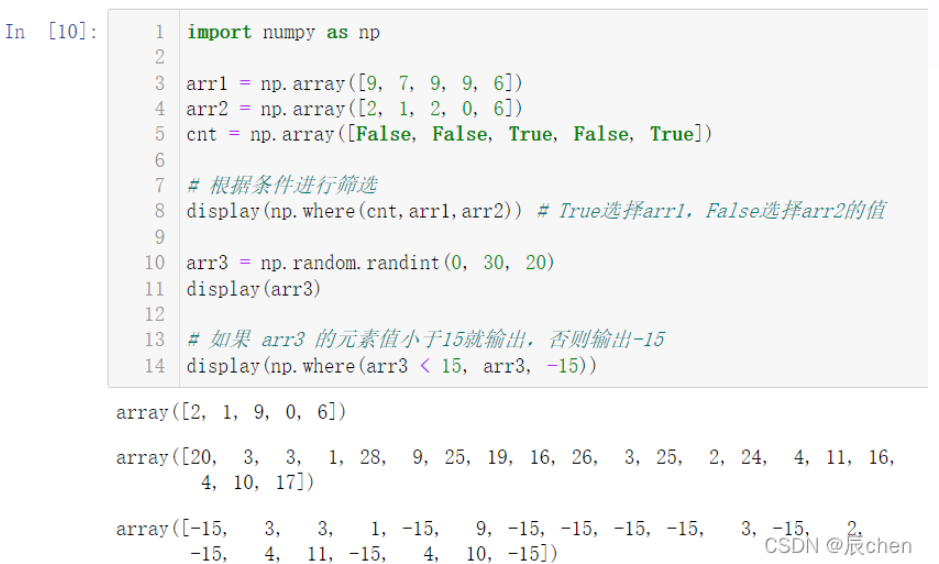## 3.3排序方法

🚩NumPy中还提供了排序方法，排序方法是就地排序，即直接改变原数组：

arr.sort()、np.sort()、arr.argsort()

```import numpy as np
arr = np.array([14, 9, 13, 13, 18, 18, 18, 7, 5, 11])
# 直接让原数组从小到大进行排序
arr.sort()
display(arr)
# 返回深拷贝排序结果
np.sort(arr)
arr = np.array([14, 9, 13, 13, 18, 18, 18, 7, 5, 11])
display(arr)
# 返回从小到大排序的索引
display(arr.argsort()) ```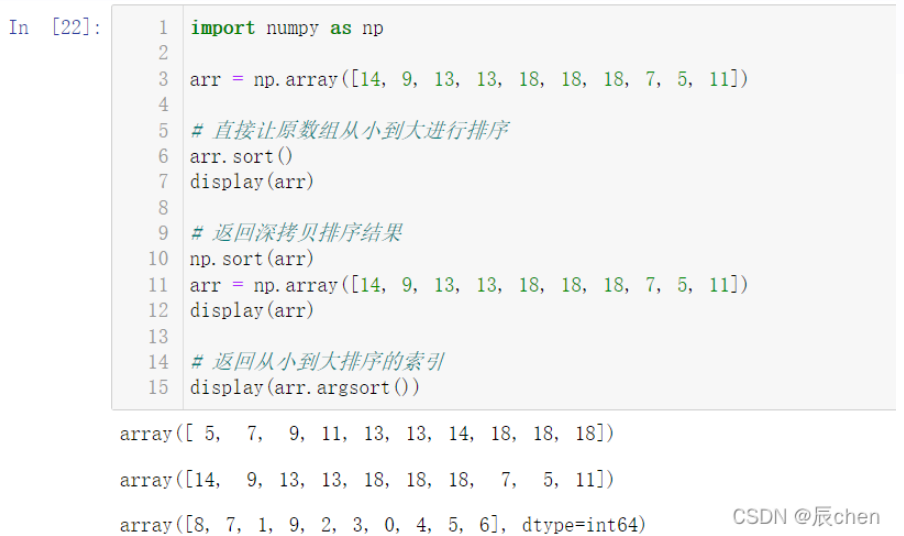## 3.4 集合运算函数

```A = np.array([6, 8, 9, 1, 4])
B = np.array([3, 6, 5, 7, 1])
# 计算交集
display(np.intersect1d(A, B))
# 计算并集
display(np.union1d(A, B))
# 计算差集
display(np.setdiff1d(A, B))```## 3.5数学和统计函数

🚩我们挑几个常用的函数进行代码演示，剩余的函数有兴趣的读者可以自行查阅用法自己演示。min、max、mean、median、sum、std、var、cumsum、cumprod、argmin、argmax、argwhere、cov、corrcoef

```import numpy as np
arr1 = np.array([5, 90, 87, 35, 23,  6, 39, 39, 99, 79, 72, 94, 97, 13, 84])
# 计算数组中的最小值
display(arr1.min())
# 计算数组中的最大值的索引
display(arr1.argmax())
# 返回大于40的元素的索引
display(np.argwhere(arr1 > 40))
# 计算数组的累加和
display(np.cumsum(arr1))
arr2 = np.random.randint(0, 10,size = (4, 5))
display(arr2)
# 计算列的平均值
display(arr2.mean(axis = 0))
# 计算行的平均值
display(arr2.mean(axis = 1))
# 协方差矩阵
display(np.cov(arr2, rowvar = True))
# 相关性系数
display(np.corrcoef(arr2, rowvar = True))```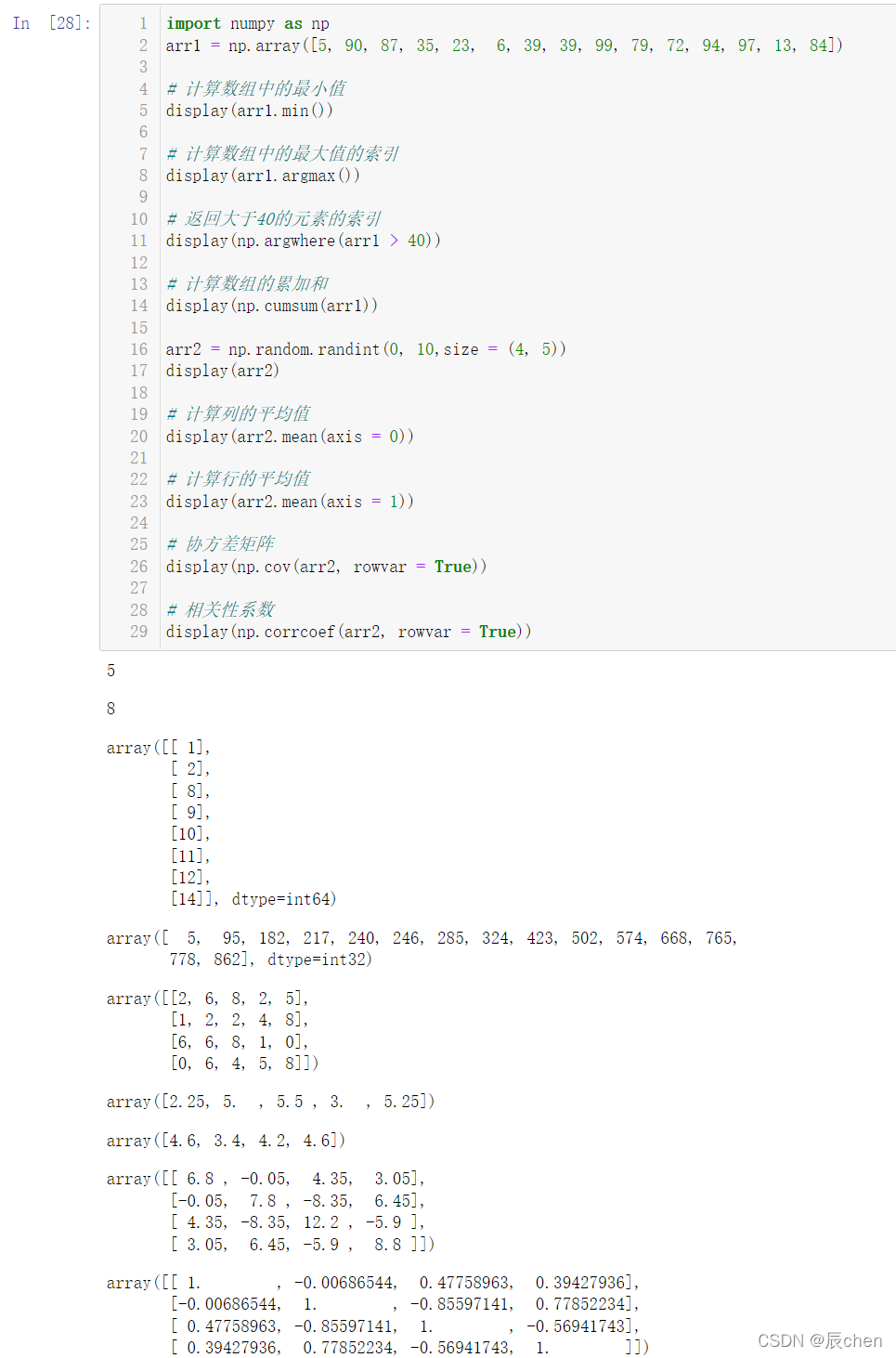# 4.矩阵运算

## 4.1 矩阵的乘法

```#矩阵的乘积(点乘)
A = np.array([[2, 1, 7],
[6, 3, 4]]) # shape(2, 3)
B = np.array([[4, 3],
[0, 9],
[-5, -8]]) # shape(3, 2)
# 第一种方法
display(np.dot(A,B))
# 第二种方法
display(A @ B) # 符号 @ 表示矩阵乘积运算
# 第三种方法
display(A.dot(B))```

## 4.2 矩阵的其他运算

```np.set_printoptions(suppress = True) # 不使用科学计数法
from numpy.linalg import inv,det,eig,qr,svd
A = np.array([[1, 2, 3],
[2, 3, 1],
[3, 2, 1]]) # shape(3, 3)
# 求逆矩阵
B = inv(A)  # B 就是 A 的逆矩阵
display(B)
display(A.dot(B))
# 求矩阵的行列式
display(det(A))```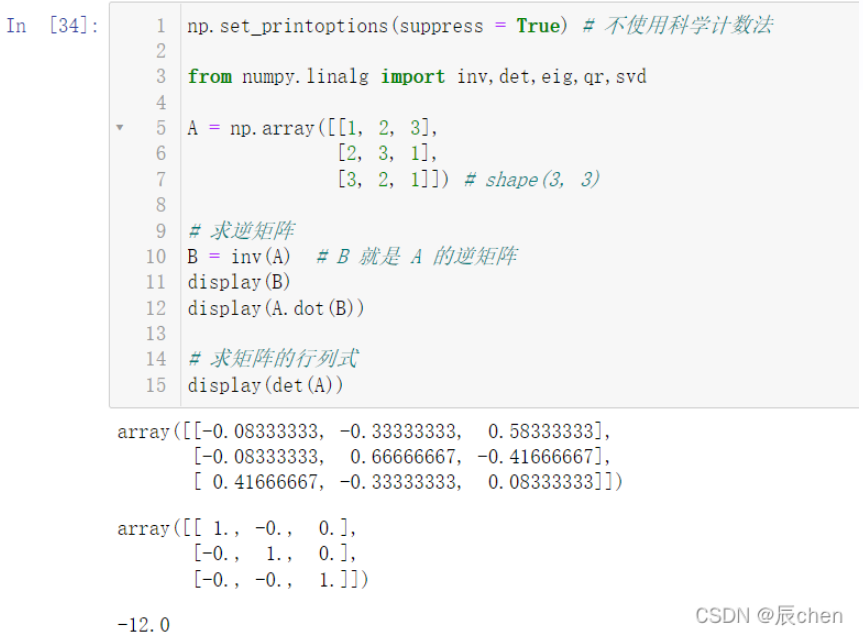# 5.训练场

## 5.1 给定一个4维矩阵，如何得到最后两维的和？（提示，指定axis进行计算）

```import numpy as np
arr = np.random.randint(0, 10, size = (2, 3, 4, 5))
display(arr)
display(arr.sum(axis = 0))  # 第0维的数据进行加和
display(arr.sum(axis = 1))  # 第1维的数据进行加和
display(arr.sum(axis = -1)) # 最后一维的数据进行加和
# 最后两维的和
# 写法一：
display(arr.sum(axis = (-1, -2)))
# 写法二：
display(arr.sum(axis = (2, 3)))```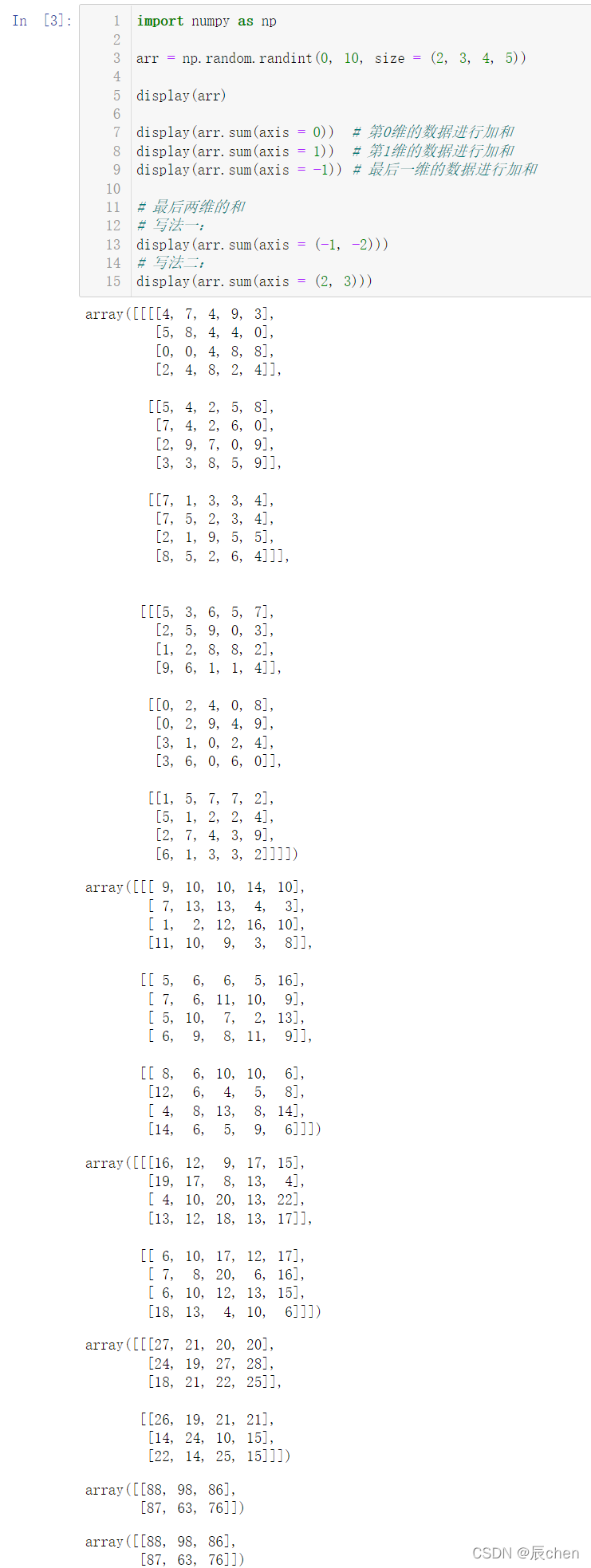## 5.2 给定数组[1, 2, 3, 4, 5]，如何得到在这个数组的每个元素之间插入3个0后的新数组？

```import numpy as np
arr1 = np.arange(1, 6)
display(arr1)
# 每个元素之间插入3个0，共插入3*4=12个0
# 再加上初始的5个值，共需要17个位置
# 创建一个长度为17的全部是0的数组
arr2 = np.zeros(shape = 17, dtype = np.int16)
display(arr2)
# 有间隔的，每4个取一个，取出数据并进行替换
arr2[::4] = arr1
display(arr2)```

## 5.3 给定一个二维矩阵（5行4列），如何交换其中两行的元素（提示：任意调整，花式索引）？

```import numpy as np
arr = np.random.randint(0, 100, size = (5, 4))
display(arr)
# 使用花式索引进行交换
arr = arr[[0, 2, 1, 3, 4]]
display(arr)```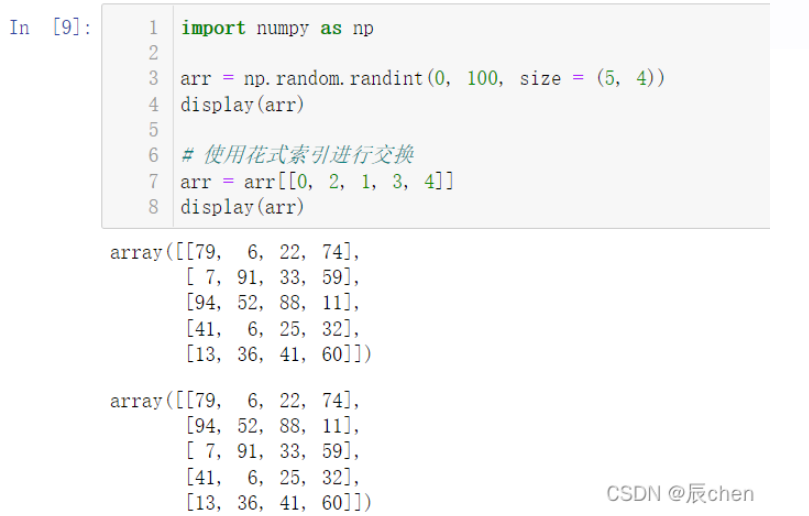### 最后编辑：2022/8/26编辑：xuanyun